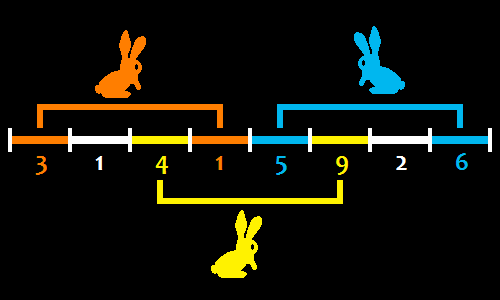### Problem Statement

Bunnies like programming and they often practice for contests.

There is a special computer named B-Computer, which all bunnies are eager to use. All bunnies want to solve a difficult problem using B-Computer. Because they type very fast, each of them wants to solve the problem according to the following process that consists of 3 stages (no delay is allowed between subsequent stages):
1. Use B-Computer for exactly one time unit.
2. Think and calculate on paper for exactly k time units, not using B-Computer.
3. Use B-Computer for exactly one time unit again to complete.
B-Computer cannot be used by more than one bunny at the same time, but when a bunny is thinking and calculating on paper, another bunny may use B-Computer.

A day is divided into a number of equal time units, and each time unit has an associated positive integer value called preference. You are given a int[] preference, which contains the preference values for a day. The number of elements in preference is the number of time units in the day, and the i-th element of preference is the preference of the i-th time unit.

Bunnies want to design a B-Computer schedule for a single day so that the sum of preferences of time units in which one of them uses B-Computer is maximized. The schedule must be such that each bunny uses B-computer exactly as described above and both time units at which the same bunny uses B-computer are in the same day. Return the maximum possible sum of preferences. You can assume that there are infinitely many bunnies.

### Definition

 Class: BunnyComputer Method: getMaximum Parameters: int[], int Returns: int Method signature: int getMaximum(int[] preference, int k) (be sure your method is public)

### Constraints

-preference will contain between 1 and 50 elements, inclusive.
-Each element of preference will be between 1 and 1,000,000, inclusive.
-k will be between 1 and 50, inclusive.

### Examples

0)

 `{ 3, 1, 4, 1, 5, 9, 2, 6 }` `2`
`Returns: 28`
 The sum is maximized when three bunnies use B-Computer as follows: One bunny uses B-Computer during the 1-st time unit and again during the 4-th time unit. One bunny uses B-Computer during the 3-rd time unit and again during the 6-th time unit. One bunny uses B-Computer during the 5-th time unit and again during the 8-th time unit.1)

 `{ 3, 1, 4, 1, 5, 9, 2, 6 }` `1`
`Returns: 31`
2)

 `{ 1, 2, 3, 4, 5, 6 }` `3`
`Returns: 14`
3)

 `{ 487, 2010 }` `2`
`Returns: 0`
 No bunnies can use B-Computer.

#### Problem url:

http://www.topcoder.com/stat?c=problem_statement&pm=11157

#### Problem stats url:

http://www.topcoder.com/tc?module=ProblemDetail&rd=14240&pm=11157

lyrically

#### Testers:

PabloGilberto , ivan_metelsky , soul-net

#### Problem categories:

Simple Math, Simple Search, Iteration